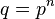# Regular p-group

This article defines a group property: a property that can be evaluated to true/false for any given group, invariant under isomorphism
View a complete list of group properties
VIEW RELATED: Group property implications | Group property non-implications |Group metaproperty satisfactions | Group metaproperty dissatisfactions | Group property satisfactions | Group property dissatisfactions

## Definition

Suppose$p$ is a prime number. A p-group$G$ (i.e., a group where the order of every element is a power of$p$) is termed a regular$p$-group if it satisfies the following equivalent conditions:

1. For every$a,b \in G$, there exists$c \in [\langle a,b\rangle, \langle a,b \rangle]$ such that$a^pb^p = (ab)^pc^p$.
2. For every$a,b \in G$, there exist$c_1,c_2, \dots, c_k \in [\langle a,b\rangle, \langle a,b \rangle]$ such that$a^pb^p = (ab)^pc_1^pc_2^p \dots c_k^p$.
3. For every$a,b \in G$ and every natural number$n$, there exist$c_1,c_2, \dots, c_k \in [\langle a,b\rangle, \langle a,b \rangle]$ such that$a^qb^q = (ab)^qc_1^qc_2^q \dots c_k^q$ where$q = p^n$.

The term regular p-group is typically used only for finite p-groups.

## Metaproperties

Metaproperty name Satisfied? Proof Statement with symbols
subgroup-closed group property Yes regular p-group property is subgroup-closed If$p$ is prime,$G$ is a regular$p$-group, and$H$ is a subgroup of$G$, then$H$ is a regular$p$-group.
quotient-closed group property Yes regular p-group property is quotient-closed If$p$ is prime,$G$ is a regular$p$-group,$H$ is a normal subgroup of$G$, and$G/H$ is the corresponding quotient group, then$G/H$ is also a regular$p$-group.
finite direct product-closed group property No regular p-group property is finite direct product-closed It is possible to have a prime number$p$ and regular$p$-groups$G_1,G_2$ such that the external direct product$G_1 \times G_2$ is not a regular$p$-group.
2-local group property Yes regular p-group property is 2-local Suppose$p$ is a prime number and$G$ is a group such that for all$a,b \in G$,$\langle a,b \rangle$ is a regular$p$-group, then$G$ itself is a regular$p$-group.